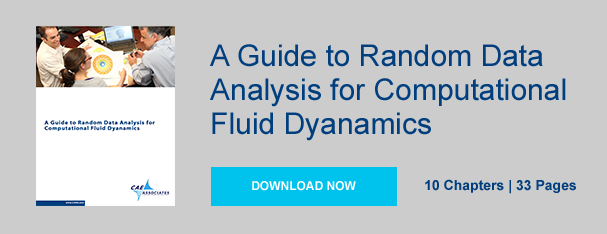you are here:/ News & Insights / Engineering Advantage Blog / Selecting a Target Transient Time Step in a CFD Analysis

# Selecting a Target Transient Time Step in a CFD AnalysisMarch 27, 2015 By: Jonathan Dudley

A frequent question asked when preparing to conduct a transient CFD analysis is: What time step should I choose for my simulation? As with any engineering method, one must always consider the tradeoff between accuracy and computational cost when making this decision. Smaller time steps will typically yield more accuracy and stability, at the expense of longer run-times and increased computational cost.

Open cavity flow

## transient time step 1.pngFigure 1 illustrates an open cavity flow common in external aerodynamics. The length of the cavity is 76.2mm and the free stream velocity is taken as 415 m/s. A traditional approach for selecting a target time step relies on choosing the appropriate length and velocity scale for the problem of interest. In this problem, it could be argued that the appropriate length scale is the length of the cavity (L). The appropriate velocity scale is likely to be the convective velocity of the shear layer, which is significantly slower than the free stream velocity. For a conservative estimate, the free stream velocity may be used.  If we take a principles first approach, we may estimate the average length of time it takes for a vortical structure to travel from the leading to trailing edge of the cavity. Research indicates the convective velocity of the shear layer is approximately 50% of the free stream: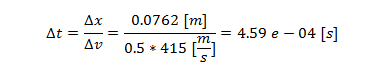From here, we require the flow to take “N” divisions (as we do not want the flow to leap from the leading to trailing edge in one iteration) in order to achieve time accurate results. In essence, to choose our time step (dt) we employ: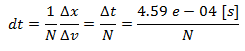where N is a integer value commonly no smaller than 10 and as high as 200 or more! Let’s assume we further understand this cavity to resonant at a peak frequency of 8 [khz]. In order to resolve this frequency one may consider the period of the frequency of interest and divide it into intervals similar as above: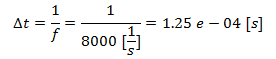We then require we take “N” subdivisions as before, such that resulting time step be of the form: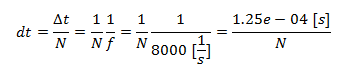In this case for fixed N, it is easy to see that resolving the frequency is a more demanding criterion,  as it requires the smaller time step.

A third option for selecting time step size will rely on the well-known Courant-Fredrichs-Lewy condition (CFL number) where we require that: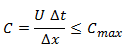That is to say, while choosing our time step, we will consider how many grid-cells we will allow our flow to pass through by monitoring the RMS and Max Courant number. To ensure an unconditionally stable time accurate solution, we would require globally that the max Courant number be less than or equal to unity (for explicit solvers, implicit solvers the max Courant number may be significantly higher). For simplicity, we will assume our cavity is discretized uniformly with 100 grid points over L. To ensure a Max Courant number of 1 we require: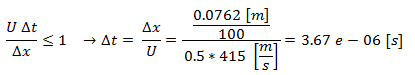Taking N=20 this would result in the following recommend range of target time steps:

Length And Velocity Scale: dt = 2.30e-05 [s]

Frequency Calculation: dt = 6.25e-06 [s]

Courant Number: dt = 3.67e-06 [s]

To ensure we temporally resolve the desired frequency and other associated physics relevant to the problem we would choose a time step between these values. Further, we would need to monitor our residuals and monitors during the simulation to ensure our target time step is offering the best balance between accuracy and computational cost.